Next: Maximum Likelihood estimation Up: Probabilistic ICA model Previous: Probabilistic ICA model

## Uniqueness

[Rao, 1969] extends the standard factor analysis model such that the common and specific variables are independent non-degenerate random variables and examines the implication for the minimum rank of the mixing matrix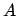in equation 2. Earlier work [Rao, 1966] characterised the multivariate normal distribution through the non-uniqueness of its linear structure, a result which within the ICA literature has been restated as the limitation that only one Gaussian source process, at most, may contribute to the observations for the ICA model to be estimable [Comon, 1994,Hyvärinen et al., 2001]. Here, a vector variable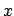is said to have a linear structure if it can be decomposed as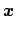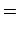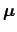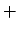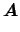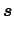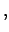(3)

where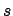is a vector of non-degenerate statistically independent one dimensional random variables andis a matrix of full column rank. The linear structure is said to be essentially unique if all the linear decompositions are equivalent in the sense that if the vector variableallows for two structural representations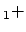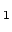and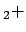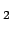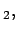(4)

then every column ofis a multiple of some column ofand vice versa, i.e. the two matrices are identical modulo scaling and permutation. This again has been noted as a standard restriction within the ICA framework [Comon, 1994].

The main result in [Rao, 1969] is a decomposition theorem that states that ifis a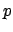-variate random variable with a linear structure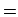where all the elements ofare non-Gaussian variables, then there does not exist a non-equivalent linear structure involving the same number or a smaller number of structural variables than that of.

Furthermore, ifis a-vector random variable with a linear structurethencan be decomposedwhereandare independent,is non-Gaussian and has an essentially unique linear structure andis-variate normal with a non-unique linear structure.

The proofs involve the characteristic functions of the vector random variablesandand as such these results are applicable only if the number of observations (i.e. voxels) is sufficiently large to accurately reflect the distribution of these quantities.

The results show, however, that conditioned on knowing the number of source signals contained in the data and under the assumption that the data are generated according to equation 2, i.e. a linear mixture of independent non-Gaussian source signals confounded by Gaussian noise, there is no non-equivalent decomposition into this number of independent non-Gaussian random variables and an associated mixing matrix; the decomposition into independent components is unique, provided we do not attempt to extract more than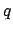source signals from the data.Next: Maximum Likelihood estimation Up: Probabilistic ICA model Previous: Probabilistic ICA model
Christian F. Beckmann 2003-08-05